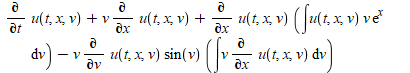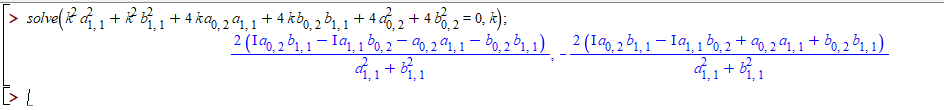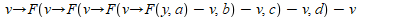## How to separate a maths function from a maple expr...

Please I will like to know if there exists any command to isolate the known regular mathematical functions from an expression. For example, given the equationI need a means of isolating (ex & sin(v)) from the above expression

Thanks

## export image with minimum size...

How can we export image with minimum size? I plot the solution and then export the image as an .eps file. But when I try to generate the generate the pdf file on Latex, the file size is too large. But when I plot the solutions on Matlab and export the images as .eps file, then Latex generate pdf file with samll size. Why Maple generate images of large sizes?

solution.mw

## Maple Calculus Study Guide displays in Maple notat...

I am using the Calculus Study Guide and the mathematical notation is half Maple notation and then half typeset notation. I have tried to tweak with Tools>Options>Display settings but it stays the same

For example:

{a[n]}[n = n]^infinity;, where
"a[n]"

is the general term for the sequence, and
"n"

is the starting index.
The notation is often shortened to {a[n]}; to save printing costs.

...and the math notation past the word "where" is in typeset notation but changed to Maple Notation when I cut and pasted.

## How to use sequence in a procedure...

Please how can I define two sequences in a procedure with two arguments?

ans:= Array([seq( [j, doCalc(j, u)], j=-2..0, 0.006, u=1..334)]):

The procedure is doCalc(j,u)  and I received this error: invalid input: seq expects between 1 and 3 arguments, but received 4

Find attached my complete code.Seq_Proc.mw

## How to replace a row of a matrix with the row of a...

How to replace 7th-row(second last) of the matrix H116 (see eq. (14)) with the 1st-row of the matrix H16 (see eq. (15)) and create a new matrix of size same as H116?

matrixop.mw

## Variables in procedures....

Hi! Can anyone show me a quick example of a procedure with local and global variables?!? It seems that i'm not getting the hang of it, because i keep receiving "unable to parse" messages.

## During evaluating function...

During evaluating psi0 (see eq, (7))why we need two values of f (i.e., f,0 and f,3)? I asked one of my seniors and according to him these values are arbitrary. Can anyone explain why we need two values and why f,0 and f,3?

## HPM error with different do and end loop for same...

Dear maple user,

I have codes for Differential equations while applying one do and end loop i am able to plot the graph of G(x) while same problem with other way of applying do and end loop i am unable to plot. whats wrong with do and end loop. These are codes available in maple primes . while combining i am unable to plot .

any one resolve it.

restart:
with(DETools):
with(plots):
with(IntegrationTools):
de0 := {
(1-p)*(diff(f(x),x,x,x))+p*(diff(f(x),x,x,x)+(1/2)*f(x)*(diff(f(x),x,x))),
(1-p)*(diff(g(x),x\$2))/Pr+p*((diff(g(x),x\$2))/Pr+(1/2)*f(x)*(diff(g(x),x)))}:

ibvc0 := {f(0),(D(f))(0),(D(f))(5)-1,g(0)-1,g(5)}:
n:=2:

F := unapply( add(b[k](x)*p^k,k=0..n), x ):
G := unapply( add(c[k](x)*p^k,k=0..n), x ):

de := map( series, eval( de0, {f=F,g=G} ), p=0, n+1 ):

for k from 0 to n do

if k = 0 then
ibvc := expand( eval[recurse]( ibvc0, {f=F,g=G,p=0} ) ):
else
ibvc := { b[k](0), D(b[k])(0), (D@@2)(b[k])(0), c[k](0), D(c[k])(0) }:
end if:

sys := simplify( map( coeff, de, p, k ) ) union ibvc:
soln := dsolve( sys ):

b[k] := unapply( eval( b[k](x), soln ), x ):
c[k] := unapply( eval( c[k](x), soln ), x ):

end do:

'F(x)' = F(x)+O(p^(n+1)):
'G(x)' = G(x)+O(p^(n+1)):

Pr:=1:
plot(eval(G(x), p = 1), x = 0 .. 5, color = blue):
###### Same problem with other  way of do and and end loop unable to plot with G(x)
restart:
with(DETools):
with(plots):
with(IntegrationTools):
Pr:=1:
de1 := (1-p)*(diff(f(x), `\$`(x, 3)))+p*(diff(f(x), `\$`(x, 3))+(1/2)*f(x)*(diff(f(x), `\$`(x, 2))));
de2 := (1-p)*(diff(g(x), `\$`(x, 2)))/Pr+p*((diff(g(x), `\$`(x, 2)))/Pr+(1/2)*f(x)*(diff(g(x), x)));
ibvc := f(0), (D(f))(0), (D(f))(5)-1, g(0)-1, g(5); n := 2; F := unapply(add(b[k](x)*p^k, k = 0 .. n), x); G := unapply(add(c[k](x)*p^k, k = 0 .. n), x);
DE1 := series(eval(de1, f = F), p = 0, n+1);
DE2 := series(eval(de2, g = G), p = 0, n+1);
CO := map(coeffs, eval([ibvc], f = F), p); CT := map(coeffs, eval([ibvc], g = G), p);

for k from 0 to n do IBVC1 := select(has, C*T, c[k]); slv := dsolve({coeff(DE2, p, k), op(IBVC1)}); c[k] := unapply(rhs(slv), x) end do;
G(x) = G(x)+O(p^(n+1));
plot(eval(G(x), p = 1), x = 0 .. 5);

## How to rectify the "Error, invalid subscript selec...

Hi, Maple users

I hope you are doing well.
Here I solved one of the ode problems by dsolve.
But I am getting  "Error, invalid subscript selector" error.
Kindly do the needful to find a solution.
Thank you.

JVB.mw

## How can i plot phase portrait? What is the problem...

I want to plot phase portrait but i get some errors. I didn't understand errors? Can anyone help me? zuhal_faz_portresi.mw

## How do I solve second order equations and obtain r...

I have been studying the solutions of the equation below using Maple and Mathematica.

Here, I want to express the solutions for k. In Maple, the solution is imaginary. As one may see below.On the other hand, if one solves that  exact equation with Mathematica, then one has real solutions. That is,

Based on the above, I have a few questions:

1. What is the correct solution for the first equation above? That is, are the solutions real or complex?

2. How may I obtain the real solutions, k_{1,2}, above using Maple?

Attached below you may find my mwfile with my doubts.

Mydoubts.mw

## I need syntax help on procedures....

Hi! I'm having doubts about the "automatic spacing" in maple. I would like to know two things:

1-can incorrect spacing ruin my code? (Ex by typing "spacebar" too many times )

2- i know that to type spacebar is obligatory after: proc()" "...end proc;    Is there any other situation in wich to use spacebar isn't optional? Thanks for your answers

## Reverse a catenation...

I want to reverse a catenated expression (eg. xxtt ->x,x,t,t). I don't know the command to achieve this. Anyone with an idea please help

Thanks

## How do i add elements from subsets?...

Hi! I've got a set of subsets S with Abeing the i-subset. I need to sum up all the x's in all the Ai's. How can this be achieved?

(ex):

sum( { {a, b, c}, {a, b, d} } )= 2a+2b+c+d

## arrow procedure...

Hello,

I am writing an arrow procedure and will like to know if there is a way to implement the following

bj := (G, y) ->  (`@`(seq((t -> u -> v -> G(u, t) - v)(args[1 + nargs - i]), i = 1 .. nargs - 2)))(y)
bj(F, y, a, b, c, d);
v:=[p, q, r, s]
the outputMy question is, how can I replace the v with each element of the list to get the following as output
F(F(F(F(y,a)-p),b)-q,c)-r,d)-s

Aany suggestion will be highly appreciated

﻿